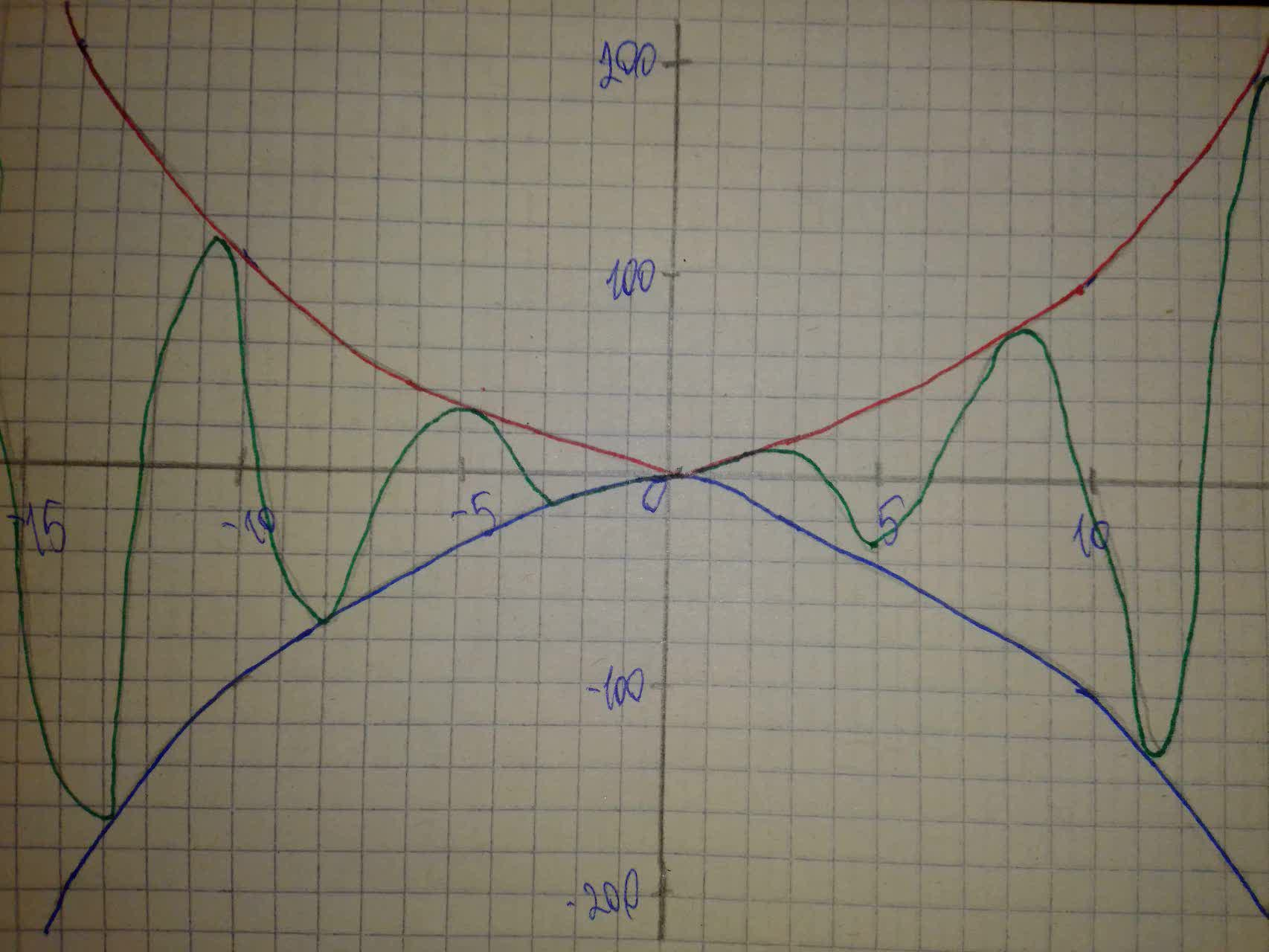# Investigate the change in the graph of a sinusoidal function of the form and=\sin x or and=\cos x when multiplied by a polynomial function. Use a graphing calculator to sketch the graphs of and=x^{2},and=−x^{2} and and=x^{2} \sin x on the same coordinate plane.texelaare 2021-08-02 Answered
Investigate the change in the graph of a sinusoidal function of the form $$\displaystyle{\quad\text{and}\quad}={\sin{{x}}}$$ or $$\displaystyle{\quad\text{and}\quad}={\cos{{x}}}$$ when multiplied by a polynomial function. Use a graphing calculator to sketch the graphs of $$\displaystyle{\quad\text{and}\quad}={x}^{{{2}}},{\quad\text{and}\quad}=−{x}^{{{2}}}$$ and $$\displaystyle{\quad\text{and}\quad}={x}^{{{2}}}{\sin{{x}}}$$ on the same coordinate plane, on the interval [-20, 20].

• Questions are typically answered in as fast as 30 minutes

### Plainmath recommends

• Get a detailed answer even on the hardest topics.
• Ask an expert for a step-by-step guidance to learn to do it yourself.Daphne Broadhurst
Here is the graph: